## Header Ads

In electromagnetism, permeability is the measure of the ability of a material to support the formation of a magnetic field within itself. In other words, it is the degree of magnetization that a material obtains in response to an applied magnetic field. Magnetic permeability is typically represented by the Greek letter μ. The term was coined in September, 1885 by Oliver Heaviside. The reciprocal of magnetic permeability is magnetic reluctivity.
In SI units, permeability is measured in the henry per metre (H m−1), or newton per ampere squared (N A−2). The permeability constant (μ0), also known as the magnetic constant or the permeability of free space, is a measure of the amount of resistance encountered when forming a magnetic field in a classical vacuum. The magnetic constant has the exact (defined) value µ0 = 4π×10−7 ≈ 1.2566370614...×10−6 H·m−1 or N·A−2).
_____________________________________________________________________________________

## Explanation

In electromagnetism, the auxiliary magnetic field H represents how a magnetic field B influences the organization of magnetic dipoles in a given medium, including dipole migration and magnetic dipole reorientation. Its relation to permeability is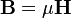$\mathbf{B}=\mu \mathbf{H}$
where the permeability μ is a scalar if the medium is isotropic or a second rank tensor for an anisotropic medium.
In general, permeability is not a constant, as it can vary with the position in the medium, the frequency of the field applied, humidity, temperature, and other parameters. In a nonlinear medium, the permeability can depend on the strength of the magnetic field. Permeability as a function of frequency can take on real or complex values. In ferromagnetic materials, the relationship between B and H exhibits both non-linearity and hysteresis: B is not a single-valued function of H, but depends also on the history of the material. For these materials it is sometimes useful to consider the incremental permeability defined as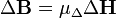$\Delta\mathbf{B}=\mu_{\Delta} \Delta\mathbf{H}$.
This definition is useful in local linearizations of non-linear material behavior, for example in a Newton-Raphson iterative solution scheme that computes the changing saturation of a magnetic circuit.
Permeability is the inductance per unit length. In SI units, permeability is measured in henries per metre (H·m−1 = J/(A2·m) = N A−2). The auxiliary magnetic field H has dimensions current per unit length and is measured in units of amperes per metre (A m−1). The product μH thus has dimensions inductance times current per unit area (H·A/m2). But inductance is magnetic flux per unit current, so the product has dimensions magnetic flux per unit area. This is just the magnetic field B, which is measured in webers (volt-seconds) per square-metre (V·s/m2), or teslas (T).
B is related to the Lorentz force on a moving charge q:$\mathbf{F} = q (\mathbf{E} + \mathbf{v} \times \mathbf{B})$.
The charge q is given in coulombs (C), the velocity v in m/s, so that the force F is in newtons (N):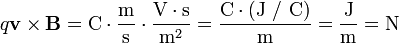$q \mathbf{v} \times \mathbf{B} = \mbox{C} \cdot \dfrac{\mbox{m}}{\mbox{s}} \cdot \dfrac{\mbox{V} \cdot \mbox{s}}{\mbox{m}^2} = \dfrac{\mbox{C} \cdot (\mbox{J / C})}{\mbox{m}} = \dfrac{\mbox{J}}{\mbox{m}} = \mbox{N}$
H is related to the magnetic dipole density. A magnetic dipole is a closed circulation of electric current. The dipole moment has dimensions current times area, units ampere square-metre (A·m2), and magnitude equal to the current around the loop times the area of the loop. The H field at a distance from a dipole has magnitude proportional to the dipole moment divided by distance cubed, which has dimensions current per unit length.
___________________________________________________________________________________

## Relative permeability

Relative permeability, sometimes denoted by the symbol μr, is the ratio of the permeability of a specific medium to the permeability of free space given by the magnetic constant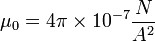$\mu_0 = 4 \pi \times 10^{-7} \frac{N}{A^2}$: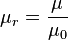$\mu_{r} = \frac{\mu}{\mu_{0}}$.
In terms of relative permeability, the magnetic susceptibility is:
χm = μr − 1.
χm, a dimensionless quantity, is sometimes called volumetric or bulk susceptibility, to distinguish it from χp (magnetic mass or specific susceptibility) and χM (molar or molar mass susceptibility).

## Diamagnetism

Diamagnetism is the property of an object which causes it to create a magnetic field in opposition of an externally applied magnetic field, thus causing a repulsive effect. Specifically, an external magnetic field alters the orbital velocity of electrons around their nuclei, thus changing the magnetic dipole moment in the direction opposing the external field. Diamagnets are materials with a magnetic permeability less than μ0 (a relative permeability less than 1).
Consequently, diamagnetism is a form of magnetism that is only exhibited by a substance in the presence of an externally applied magnetic field. It is generally a quite weak effect in most materials, although superconductors exhibit a strong effect.